# Graph It!

3.6
452 reviews
50K+Everyone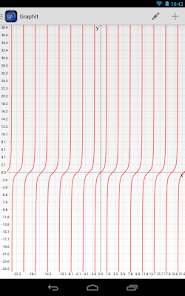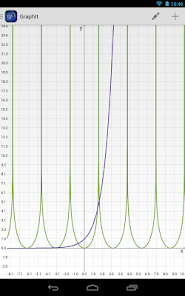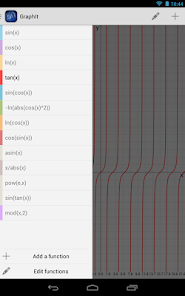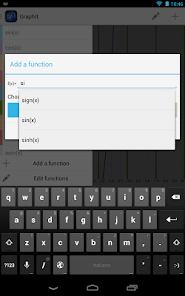GraphIt is a utility that is used to display on a Cartesian plane any mathematical function, which can be f(x)=2, f(x)=2*x, f(x)=sin(x) ecc... The management function is entrusted to you: you can add more or modify existing ones.

The integration of functions is facilitated by self-completion of statements, also expressions of the interpreter is very flexible and allows the inclusion of any function: even the most complex are recognized and interpreted correctly.
The main functions recognized are: abs(x), acos(x), acosh(x), asin(x), asinh(x), atan(x), avg(x1,x2,...,xN), ceil(x), combin(x,y), cos(x), cosh(x), e(), exp(x), fact(x), floor(x), lg(x), ln(x), log(x,y), max(x1,x2,...,mN), min(x1,x2,...,xN), mod(x,y), pi(), pow(x,y), rand(), sign(x), sin(x), sinh(x), sqrt(x), sum(x1,x2,...,xN), tan(x), tanh(x), toDeg(x), toRad(x).

The graph allows an accurate view of the trend of function at any point in the plane. You can zoom and pan through the classical gestures.

There is also a tool which can calculate the value of the functions included, given the value of x (via a number or expression).
Updated on
Apr 23, 2018

## Data safetyNo information available

## Ratings and reviews

3.6
404 reviews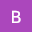Bhogavalli Maruthi
January 11, 2022
Best app for geometry drawings . We can quick refer .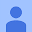November 13, 2013
Its brillant compared to other graphing apps. The only feature it lacks is the ability to plot graphs where both x and y are on the same side od equation. But its 5 stars for the design.
13 people found this review helpful